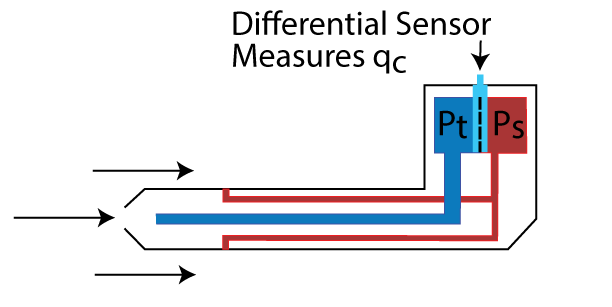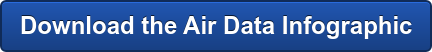Air data is a measurement of the air mass surrounding an airplane. The two physical characteristics measured are pressure and temperature. Air data is acquired through various sensors on the aircraft and is used to calculate altitude, speed, rate of climb or decent, and angle-of-attack or angle-of-sideslip.

## What pressure data is measured?

The pressure measurements consist of static pressure (Ps) and total pressure (Pt).

Definitions:

• Static Pressure (Ps) is the absolute pressure of still air surrounding the aircraft. This is the barometric pressure at the altitude where the aircraft is traveling and is independent of any pressure disturbances caused by the motion of the aircraft.
• Total Pressure (Pt) is the sum of the local atmospheric pressure (Ps) and the impact pressure (Qc) caused by the aircraft's motion through the air.
• Impact Pressure (Qc) is the pressure a moving stream of air produces against a surface that brings part of the moving stream to rest. It is the difference between the total pressure (Pt) and the static pressure (Ps).

These pressure properties are related by the formula: Qc = Pt - Ps

Ps and Pt are acquired by one or more pitot static probes on the aircraft body. Qc is calculated from these values.

Ps is used to calculate the altitude of the aircraft.

Qc is used to calculate the speed of the aircraft.## How is altitude determined?

Altitude is derived from a series of equations using the static pressure. Altitude calculations are based on a "standard atmosphere," which assumes a known relationship between pressure, temperature and atmospheric density. Generally, the lower the static pressure, the higher the altitude.

## Why is temperature measured?

Temperature is measured in order to calculate true airspeed (the actual speed of the plane through air) from indicated airspeed and temperature.  A temperature probe on the body of the aircraft acquires temperature values. Pressure and temperature data sensing is sometimes combined in the same sensor.

Indicated airspeed (IAS), true airspeed (TAS) and Mach number are versions of an aircraft’s speed and have a temperature component incorporated.

Definitions:

• Indicated Airspeed (IAS): The speed of an aircraft relative to the surrounding air. It is uncorrected for any installation or instrument errors. Indicated airspeed equals true airspeed at standard sea level conditions only, and it is a function of impact pressure (Qc).
• True Airspeed (TAS): Indicated airspeed corrected for nonstandard temperatures that can be determined using Mach number and total temperature. It is the actual aircraft speed through the air mass.
• Mach Number: The ratio of true airspeed and the speed of sound in the surrounding air. The speed of sound is proportional to the square root of the average temperature. Mach number is calculated using the ratio of impact pressure (Qc) to static pressure (Ps).

Reference: Rosemount Aerospace, Air Data Handbook